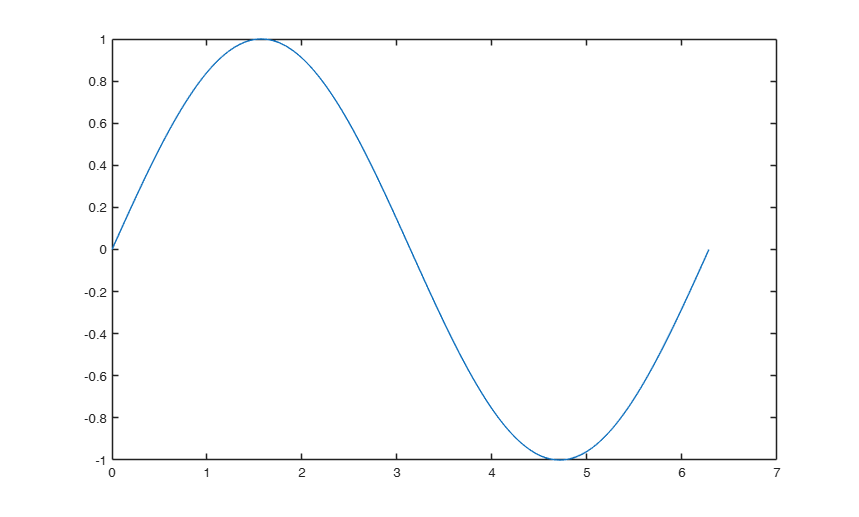# cabc

cabc closes all open figures except the one in foreground (the current)

## Description

 cabc() Plot sin, cos and atan in the interval 0 2pi.

## Examples

expand all

###Plot sin, cos and atan in the interval 0 2pi.

x = 0:pi/1000:2*pi;
plot(x,sin(x));
figure
plot(x,cos(x));
figure
plot(x,atan(x))
% Now highlight a figure and then digit
cabc
% All the other figures but the one which has been selected will be
% closed## Related Examples

expand all

### Use of routine cabc inside GUI brushRES.

brushRES

## Output Arguments

Tufte E.R. (1983), "The visual display of quantitative information", Graphics Press, Cheshire.